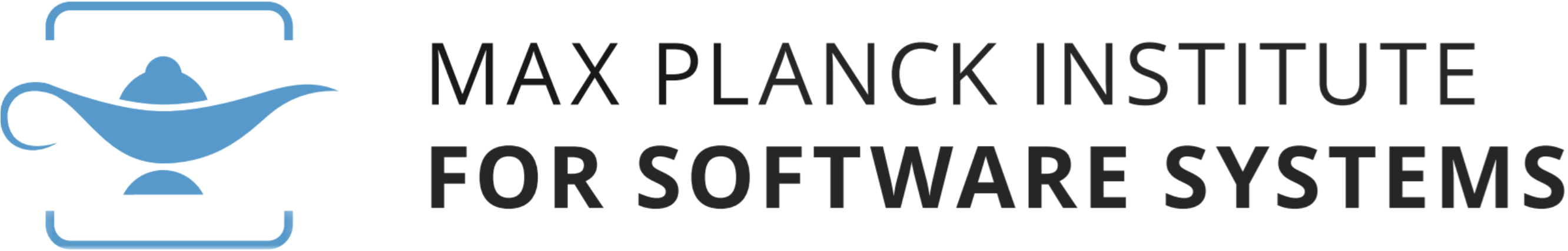## Research Spotlight: From Newton to Turing to cyber-physical systemsIn 1937, a young Englishman by the name of Alan M. Turing published a paper with the obscure title On computable numbers, with an application to the Entscheidungsproblem in the Proceedings of the London Mathematical Society. In doing so, he arguably laid the mathematical foundations of modern computer science. Turing’s seminal contribution was to show that the famous Entscheidungsproblem, formulated by the great German mathematician David Hilbert several years earlier, could not be solved: more precisely, Turing proved (in modern parlance) that the problem of determining whether a given computer program halts could not be done algorithmically—in other words that the famous Halting Problem is undecidable.

Although seemingly at the time a rather esoteric concern, the Halting Problem (and related questions) have dramatically gained in importance and relevance in more contemporary times. Fast forward to the 21st Century: nowadays, it is widely acknowledged that enabling engineers, programmers, and researchers to automatically verify and certify the correctness of the computer systems that they design is one of the Grand Challenges of computer science. In increasingly many instances, it is absolutely critical that the software governing various aspects of our daily lives (such as that running on an aircraft controller, for example) behave exactly as intended, lest catastrophic consequences ensue.

### What classes of infinite-state programs can be analyzed algorithmically?

Researchers at the Foundations of Algorithmic Verification group are investigating what classes of infinite-state programs can, at least in principle, be fully handled and analyzed algorithmically by viewing computer programs abstractly as dynamical systems, and they seek to design exact algorithms enabling one to fully analyse the behaviour of such systems. In particular, they are presently tackling a range of central algorithmic problems from verification, synthesis, performance, and control for linear dynamical systems, drawing among others on tools from number theory, Diophantine geometry, and algebraic geometry, with the overarching goal of offering a systematic exact computational treatment of various important classes of dynamical systems and other fundamental models used in mathematics, computer science, and the quantitative sciences. Some of their achievements include several decidability and hardness results for linear recurrence sequences, which can be used to model simple loops in computer programs, answering a number of longstanding open questions in the mathematics and computer science literature.

In a series of recent papers [1, 2],  they have attacked the so-called Zero Problem for linear differential equations, i.e., the question of determining algorithmically whether the unique solution to a given linear differential equation has a zero or not. Such equations, which go back as far as Newton, are ubiquitous in mathematics, physics, and engineering; they are also particularly useful to model cyber-physical systems, i.e., digital systems that evolve in and interact with a continuous environment. In their work, they obtained several important partial results: if one is interested in the existence of a zero over a bounded time interval, then it is possible to determine this algorithmically, provided that a certain hypothesis from the mathematical field of number theory, known as Schanuel’s Conjecture, is true. They were also able to partially account for the fact that the Zero Problem has hitherto remained open in full generality: indeed, if one were able to solve it in dimension 9 (or higher), then in turn this would enable one to solve various longstanding hard open problems from a field of mathematics known as Diophantine approximation. In doing so, they therefore exhibited surprising and unexpected connections between the modelling and analysis of cyber-physical systems and seemingly completely unrelated deep mathematical theories dealing with questions about whole numbers.

#### References

 Ventsislav Chonev, Joel Ouaknine, and James Worrell. On recurrent reachability for continuous linear dynamical systems. In Proceedings of the 31st Annual ACM/IEEE Symposium on Logic in Computer Science (LICS), 2016.

 Ventsislav Chonev, Joel Ouaknine, and James Worrell. On the Skolem Problem for continuous linear dynamical systems. In Proceedings of the 43rd International Colloquium on Automata, Languages, and Programming (ICALP), 2016.